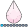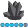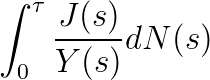JacobSimonsen
Barite | Level 11

## LaTeX equation in a title

Now and then when I create graphs I have the need of specifying a mathematical formulae either in the title or in the axis-label. My question is therefore is possible to somehow specify a LaTeX equation directly in the title statement. Something like

title1 font=??   '\int_{0}^{\infty} \frac{1}{Y(s)}dN(s)';

proc gplot data=...;

plot a*b;

run;

I most often use the device=pslepsf so that I get a epsfile, which may make the problem easier.

I hope someone have had the same problem and found a good solution. Thank you.

4 REPLIES 4AncaTilea
Pyrite | Level 9

## Re: LaTeX equation in a title

Hi.

You will probably have to use Unicode value for whatever symbols you want to use.

Could you provide a picture of the equation you would like displayed?

Anca.JacobSimonsen
Barite | Level 11

## Re: LaTeX equation in a title

This is the equation I would have i the titlethough maybe together with some more text.

## Re: LaTeX equation in a title

I would just take that image and annotate it onto the graph!I don't think there's going to be a graceful/robust way to have SAS draw equations like this (I could be wrong, of course!)JacobSimonsen
Barite | Level 11

## Re: LaTeX equation in a title

You are probably right RobertAllisson, it is perhaps most easy to just include the image it self with the annotate facility.

My idea was though not to make SAS do the equation. But rather if it could use some kind of latex-plugin which produce some postscript code. And this code could perhaps be included into the  epsfile drawn by proc gplot. If possible, then it would give a higher quality since the equation don't need to be converted to some other format.

Discussion stats
• 4 replies
• 1284 views
• 3 likes
• 3 in conversation